# Mean State

Period Mean (original grids) [Watt m-2]
Model Period Mean (intersection) [Watt m-2]
Model Period Mean (complement) [Watt m-2]
Benchmark Period Mean (intersection) [Watt m-2]
Benchmark Period Mean (complement) [Watt m-2]
Bias [Watt m-2]
RMSE [Watt m-2]
Phase Shift [months]
Bias Score 
RMSE Score 
Seasonal Cycle Score 
Spatial Distribution Score 
Interannual Variability Score 
Overall Score 
Benchmark [-] 38.3
CLM5PHSOFF [-] 34.9 34.6 0.00 39.6 15.5 -4.88 11.3 1.59 0.33 0.36 0.75 0.98 0.54 0.55
CLM5PHSON [-] 33.9 33.6 0.00 39.6 15.5 -5.32 11.4 1.57 0.31 0.37 0.75 0.98 0.53 0.55
Period Mean (original grids) [Watt m-2]
Model Period Mean (intersection) [Watt m-2]
Model Period Mean (complement) [Watt m-2]
Benchmark Period Mean (intersection) [Watt m-2]
Benchmark Period Mean (complement) [Watt m-2]
Bias [Watt m-2]
RMSE [Watt m-2]
Phase Shift [months]
Bias Score 
RMSE Score 
Seasonal Cycle Score 
Spatial Distribution Score 
Interannual Variability Score 
Overall Score 
Benchmark [-] 52.3
CLM5PHSOFF [-] 46.4 46.9 0.00 52.8 14.1 -6.00 13.6 1.29 0.43 0.36 0.84 0.99 0.54 0.59
CLM5PHSON [-] 45.3 45.8 0.00 52.8 14.1 -7.07 13.8 1.27 0.40 0.38 0.85 0.99 0.53 0.59
Period Mean (original grids) [Watt m-2]
Model Period Mean (intersection) [Watt m-2]
Model Period Mean (complement) [Watt m-2]
Benchmark Period Mean (intersection) [Watt m-2]
Benchmark Period Mean (complement) [Watt m-2]
Bias [Watt m-2]
RMSE [Watt m-2]
Phase Shift [months]
Bias Score 
RMSE Score 
Seasonal Cycle Score 
Spatial Distribution Score 
Interannual Variability Score 
Overall Score 
Benchmark [-] 39.3
CLM5PHSOFF [-] 37.0 37.0 0.00 40.4 16.6 -3.46 12.5 1.95 0.41 0.36 0.70 0.93 0.60 0.56
CLM5PHSON [-] 36.6 36.6 0.00 40.4 16.6 -3.70 12.2 1.81 0.40 0.38 0.72 0.91 0.58 0.56
Period Mean (original grids) [Watt m-2]
Model Period Mean (intersection) [Watt m-2]
Model Period Mean (complement) [Watt m-2]
Benchmark Period Mean (intersection) [Watt m-2]
Benchmark Period Mean (complement) [Watt m-2]
Bias [Watt m-2]
RMSE [Watt m-2]
Phase Shift [months]
Bias Score 
RMSE Score 
Seasonal Cycle Score 
Spatial Distribution Score 
Interannual Variability Score 
Overall Score 
Benchmark [-] 31.6
CLM5PHSOFF [-] 56.3 37.8 0.00 31.5 32.6 6.43 20.6 0.300 0.74 0.56 0.98 0.98 0.77 0.76
CLM5PHSON [-] 34.3 34.2 0.00 31.5 32.6 3.60 18.0 0.327 0.80 0.59 0.98 0.97 0.76 0.78
Period Mean (original grids) [Watt m-2]
Model Period Mean (intersection) [Watt m-2]
Model Period Mean (complement) [Watt m-2]
Benchmark Period Mean (intersection) [Watt m-2]
Benchmark Period Mean (complement) [Watt m-2]
Bias [Watt m-2]
RMSE [Watt m-2]
Phase Shift [months]
Bias Score 
RMSE Score 
Seasonal Cycle Score 
Spatial Distribution Score 
Interannual Variability Score 
Overall Score 
Benchmark [-] 23.5
CLM5PHSOFF [-] 29.0 28.9 0.00 25.9 15.5 2.93 11.2 1.45 0.37 0.36 0.79 0.28 0.57 0.45
CLM5PHSON [-] 27.8 27.7 0.00 25.9 15.5 2.49 11.1 1.43 0.36 0.36 0.79 0.23 0.55 0.44
Period Mean (original grids) [Watt m-2]
Model Period Mean (intersection) [Watt m-2]
Model Period Mean (complement) [Watt m-2]
Benchmark Period Mean (intersection) [Watt m-2]
Benchmark Period Mean (complement) [Watt m-2]
Bias [Watt m-2]
RMSE [Watt m-2]
Phase Shift [months]
Bias Score 
RMSE Score 
Seasonal Cycle Score 
Spatial Distribution Score 
Interannual Variability Score 
Overall Score 
Benchmark [-] 21.4
CLM5PHSOFF [-] 29.8 29.7 0.00 21.5 17.6 8.17 18.0 0.585 0.58 0.36 0.93 0.73 0.75 0.62
CLM5PHSON [-] 25.4 25.3 0.00 21.5 17.6 3.82 12.6 0.569 0.73 0.47 0.93 0.82 0.74 0.69
Period Mean (original grids) [Watt m-2]
Model Period Mean (intersection) [Watt m-2]
Model Period Mean (complement) [Watt m-2]
Benchmark Period Mean (intersection) [Watt m-2]
Benchmark Period Mean (complement) [Watt m-2]
Bias [Watt m-2]
RMSE [Watt m-2]
Phase Shift [months]
Bias Score 
RMSE Score 
Seasonal Cycle Score 
Spatial Distribution Score 
Interannual Variability Score 
Overall Score 
Benchmark [-] 30.0
CLM5PHSOFF [-] 35.2 35.2 0.00 30.5 14.9 4.72 11.9 0.972 0.65 0.46 0.87 0.97 0.68 0.68
CLM5PHSON [-] 35.3 35.3 0.00 30.5 14.9 4.16 11.4 0.960 0.67 0.47 0.87 0.97 0.67 0.69
Period Mean (original grids) [Watt m-2]
Model Period Mean (intersection) [Watt m-2]
Model Period Mean (complement) [Watt m-2]
Benchmark Period Mean (intersection) [Watt m-2]
Benchmark Period Mean (complement) [Watt m-2]
Bias [Watt m-2]
RMSE [Watt m-2]
Phase Shift [months]
Bias Score 
RMSE Score 
Seasonal Cycle Score 
Spatial Distribution Score 
Interannual Variability Score 
Overall Score 
Benchmark [-] 29.5
CLM5PHSOFF [-] 33.5 33.5 0.00 30.6 14.2 2.74 11.9 1.03 0.59 0.39 0.88 0.98 0.53 0.63
CLM5PHSON [-] 33.5 33.4 0.00 30.6 14.2 2.29 11.6 0.992 0.59 0.40 0.88 0.98 0.51 0.63
Period Mean (original grids) [Watt m-2]
Model Period Mean (intersection) [Watt m-2]
Model Period Mean (complement) [Watt m-2]
Benchmark Period Mean (intersection) [Watt m-2]
Benchmark Period Mean (complement) [Watt m-2]
Bias [Watt m-2]
RMSE [Watt m-2]
Phase Shift [months]
Bias Score 
RMSE Score 
Seasonal Cycle Score 
Spatial Distribution Score 
Interannual Variability Score 
Overall Score 
Benchmark [-] 33.0
CLM5PHSOFF [-] 33.6 33.5 0.00 32.8 37.1 0.788 17.9 0.272 0.81 0.60 0.98 0.85 0.73 0.76
CLM5PHSON [-] 32.3 32.2 0.00 32.8 37.1 -0.0780 17.2 0.288 0.82 0.61 0.98 0.85 0.73 0.77
Period Mean (original grids) [Watt m-2]
Model Period Mean (intersection) [Watt m-2]
Model Period Mean (complement) [Watt m-2]
Benchmark Period Mean (intersection) [Watt m-2]
Benchmark Period Mean (complement) [Watt m-2]
Bias [Watt m-2]
RMSE [Watt m-2]
Phase Shift [months]
Bias Score 
RMSE Score 
Seasonal Cycle Score 
Spatial Distribution Score 
Interannual Variability Score 
Overall Score 
Benchmark [-] 35.1
CLM5PHSOFF [-] 39.3 39.4 0.00 36.7 14.1 2.70 10.7 0.551 0.59 0.53 0.95 0.90 0.66 0.69
CLM5PHSON [-] 39.7 39.8 0.00 36.7 14.1 2.08 10.6 0.510 0.58 0.53 0.96 0.89 0.65 0.69
Period Mean (original grids) [Watt m-2]
Model Period Mean (intersection) [Watt m-2]
Model Period Mean (complement) [Watt m-2]
Benchmark Period Mean (intersection) [Watt m-2]
Benchmark Period Mean (complement) [Watt m-2]
Bias [Watt m-2]
RMSE [Watt m-2]
Phase Shift [months]
Bias Score 
RMSE Score 
Seasonal Cycle Score 
Spatial Distribution Score 
Interannual Variability Score 
Overall Score 
Benchmark [-] 19.8
CLM5PHSOFF [-] 22.9 22.9 0.00 20.7 10.0 2.22 10.2 1.16 0.71 0.53 0.85 0.90 0.69 0.70
CLM5PHSON [-] 22.5 22.5 0.00 20.7 10.0 1.77 10.1 1.13 0.71 0.53 0.87 0.87 0.68 0.70
Period Mean (original grids) [Watt m-2]
Model Period Mean (intersection) [Watt m-2]
Model Period Mean (complement) [Watt m-2]
Benchmark Period Mean (intersection) [Watt m-2]
Benchmark Period Mean (complement) [Watt m-2]
Bias [Watt m-2]
RMSE [Watt m-2]
Phase Shift [months]
Bias Score 
RMSE Score 
Seasonal Cycle Score 
Spatial Distribution Score 
Interannual Variability Score 
Overall Score 
Benchmark [-] 23.6
CLM5PHSOFF [-] 43.1 45.0 0.00 43.1 14.1 1.98 15.1 0.886 0.61 0.48 0.88 0.87 0.62 0.65
CLM5PHSON [-] 45.0 45.0 0.00 43.1 14.1 1.14 14.4 0.858 0.62 0.49 0.89 0.88 0.60 0.66
Period Mean (original grids) [Watt m-2]
Model Period Mean (intersection) [Watt m-2]
Model Period Mean (complement) [Watt m-2]
Benchmark Period Mean (intersection) [Watt m-2]
Benchmark Period Mean (complement) [Watt m-2]
Bias [Watt m-2]
RMSE [Watt m-2]
Phase Shift [months]
Bias Score 
RMSE Score 
Seasonal Cycle Score 
Spatial Distribution Score 
Interannual Variability Score 
Overall Score 
Benchmark [-] 64.3
CLM5PHSOFF [-] 76.7 76.6 0.00 66.2 16.5 10.3 18.7 0.559 0.56 0.57 0.95 0.99 0.55 0.70
CLM5PHSON [-] 78.4 78.2 0.00 66.2 16.5 10.2 18.6 0.552 0.56 0.57 0.95 0.99 0.54 0.70
Period Mean (original grids) [Watt m-2]
Model Period Mean (intersection) [Watt m-2]
Model Period Mean (complement) [Watt m-2]
Benchmark Period Mean (intersection) [Watt m-2]
Benchmark Period Mean (complement) [Watt m-2]
Bias [Watt m-2]
RMSE [Watt m-2]
Phase Shift [months]
Bias Score 
RMSE Score 
Seasonal Cycle Score 
Spatial Distribution Score 
Interannual Variability Score 
Overall Score 
Benchmark [-] 34.3
CLM5PHSOFF [-] 39.8 39.7 0.00 34.2 35.0 5.58 19.3 0.201 0.77 0.59 0.98 0.95 0.77 0.78
CLM5PHSON [-] 37.5 37.5 0.00 34.2 35.0 4.47 18.3 0.207 0.79 0.61 0.98 0.98 0.77 0.79
Period Mean (original grids) [Watt m-2]
Model Period Mean (intersection) [Watt m-2]
Model Period Mean (complement) [Watt m-2]
Benchmark Period Mean (intersection) [Watt m-2]
Benchmark Period Mean (complement) [Watt m-2]
Bias [Watt m-2]
RMSE [Watt m-2]
Phase Shift [months]
Bias Score 
RMSE Score 
Seasonal Cycle Score 
Spatial Distribution Score 
Interannual Variability Score 
Overall Score 
Benchmark [-] 24.8
CLM5PHSOFF [-] 34.0 34.0 0.00 27.0 14.3 7.01 11.6 0.886 0.50 0.42 0.91 0.87 0.62 0.62
CLM5PHSON [-] 33.8 33.8 0.00 27.0 14.3 6.58 11.1 0.872 0.52 0.44 0.91 0.85 0.59 0.62
Period Mean (original grids) [Watt m-2]
Model Period Mean (intersection) [Watt m-2]
Model Period Mean (complement) [Watt m-2]
Benchmark Period Mean (intersection) [Watt m-2]
Benchmark Period Mean (complement) [Watt m-2]
Bias [Watt m-2]
RMSE [Watt m-2]
Phase Shift [months]
Bias Score 
RMSE Score 
Seasonal Cycle Score 
Spatial Distribution Score 
Interannual Variability Score 
Overall Score 
Benchmark [-] 20.7
CLM5PHSOFF [-] 28.3 28.2 0.00 21.1 12.1 7.05 10.9 1.03 0.51 0.45 0.86 0.97 0.67 0.65
CLM5PHSON [-] 27.4 27.3 0.00 21.1 12.1 6.00 9.62 0.994 0.56 0.48 0.87 0.99 0.65 0.67
Period Mean (original grids) [Watt m-2]
Model Period Mean (intersection) [Watt m-2]
Model Period Mean (complement) [Watt m-2]
Benchmark Period Mean (intersection) [Watt m-2]
Benchmark Period Mean (complement) [Watt m-2]
Bias [Watt m-2]
RMSE [Watt m-2]
Phase Shift [months]
Bias Score 
RMSE Score 
Seasonal Cycle Score 
Spatial Distribution Score 
Interannual Variability Score 
Overall Score 
Benchmark [-] 20.3
CLM5PHSOFF [-] 27.2 27.2 0.00 20.6 10.6 6.66 14.7 0.644 0.56 0.43 0.92 0.81 0.69 0.64
CLM5PHSON [-] 23.7 23.7 0.00 20.6 10.6 3.05 10.4 0.622 0.68 0.53 0.92 0.90 0.67 0.70
Period Mean (original grids) [Watt m-2]
Model Period Mean (intersection) [Watt m-2]
Model Period Mean (complement) [Watt m-2]
Benchmark Period Mean (intersection) [Watt m-2]
Benchmark Period Mean (complement) [Watt m-2]
Bias [Watt m-2]
RMSE [Watt m-2]
Phase Shift [months]
Bias Score 
RMSE Score 
Seasonal Cycle Score 
Spatial Distribution Score 
Interannual Variability Score 
Overall Score 
Benchmark [-] 30.8
CLM5PHSOFF [-] 35.6 35.6 0.00 31.1 10.8 4.38 10.6 0.721 0.64 0.49 0.91 0.99 0.73 0.71
CLM5PHSON [-] 34.2 34.2 0.00 31.1 10.8 2.76 9.44 0.757 0.70 0.51 0.90 0.99 0.73 0.73
Period Mean (original grids) [Watt m-2]
Model Period Mean (intersection) [Watt m-2]
Model Period Mean (complement) [Watt m-2]
Benchmark Period Mean (intersection) [Watt m-2]
Benchmark Period Mean (complement) [Watt m-2]
Bias [Watt m-2]
RMSE [Watt m-2]
Phase Shift [months]
Bias Score 
RMSE Score 
Seasonal Cycle Score 
Spatial Distribution Score 
Interannual Variability Score 
Overall Score 
Benchmark [-] 26.8
CLM5PHSOFF [-] 27.7 27.6 0.00 27.1 15.2 0.494 8.44 2.24 0.73 0.36 0.64 0.86 0.47 0.57
CLM5PHSON [-] 27.1 27.1 0.00 27.1 15.2 0.130 8.33 2.25 0.74 0.37 0.64 0.81 0.45 0.56
Period Mean (original grids) [Watt m-2]
Model Period Mean (intersection) [Watt m-2]
Model Period Mean (complement) [Watt m-2]
Benchmark Period Mean (intersection) [Watt m-2]
Benchmark Period Mean (complement) [Watt m-2]
Bias [Watt m-2]
RMSE [Watt m-2]
Phase Shift [months]
Bias Score 
RMSE Score 
Seasonal Cycle Score 
Spatial Distribution Score 
Interannual Variability Score 
Overall Score 
Benchmark [-] 29.5
CLM5PHSOFF [-] 32.3 32.2 0.00 29.9 14.5 2.33 9.38 1.25 0.72 0.43 0.82 0.88 0.55 0.64
CLM5PHSON [-] 31.6 31.6 0.00 29.9 14.5 1.66 8.89 1.03 0.74 0.44 0.86 0.81 0.53 0.64
Period Mean (original grids) [Watt m-2]
Model Period Mean (intersection) [Watt m-2]
Model Period Mean (complement) [Watt m-2]
Benchmark Period Mean (intersection) [Watt m-2]
Benchmark Period Mean (complement) [Watt m-2]
Bias [Watt m-2]
RMSE [Watt m-2]
Phase Shift [months]
Bias Score 
RMSE Score 
Seasonal Cycle Score 
Spatial Distribution Score 
Interannual Variability Score 
Overall Score 
Benchmark [-] 28.0
CLM5PHSOFF [-] 32.4 32.3 0.00 29.7 12.6 2.46 12.3 0.810 0.56 0.47 0.91 0.93 0.61 0.66
CLM5PHSON [-] 33.1 33.1 0.00 29.7 12.6 2.96 12.0 0.745 0.57 0.48 0.92 0.93 0.58 0.66

# Temporally integrated period mean

BENCHMARK MEAN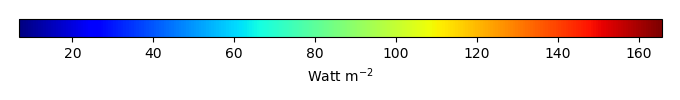MODEL MEANBIAS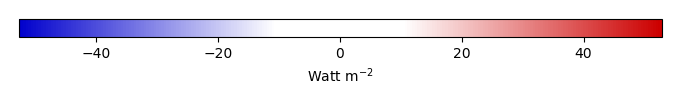BIAS SCORERMSE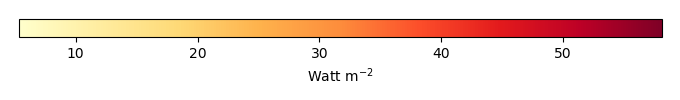RMSE SCOREBENCHMARK INTERANNUAL VARIABILITY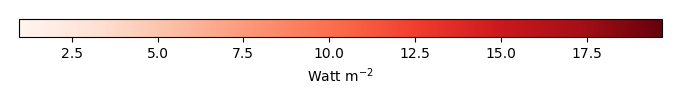MODEL INTERANNUAL VARIABILITYINTERANNUAL VARIABILITY SCOREBENCHMARK MAX MONTHMODEL MAX MONTHDIFFERENCE IN MAX MONTH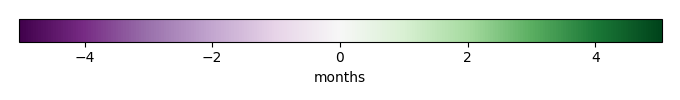SEASONAL CYCLE SCORESPATIAL TAYLOR DIAGRAMMODEL COLORS# Spatially integrated regional mean

MODEL COLORSREGIONAL MEANANNUAL CYCLEMONTHLY ANOMALYANNUAL CYCLE# All Models

BenchmarkCLM5PHSOFFCLM5PHSON# Data Information

creation_date: Mon Jun 30 23:28:16 PDT 2014

source_file: This product is generated from monthly 1 degree GEWEX SRB Radiation observations